A basic introduction to Geospatial Grids

## Basic Usage

Creating a grid from a geojson file

### Import geopandas and the geowrangler.grids module

import geopandas as gpd
import pandas as pd

from geowrangler import grids


### Load a sample geojson file into pandas

region3_gdf = gpd.read_file("../data/region3_admin.geojson")


This geopandas dataframe has the size:

length of region3_gdf: 1 row(s)

display(region3_gdf)

Reg_Code Reg_Name Reg_Alt_Name geometry
0 030000000 Region III Central Luzon MULTIPOLYGON (((120.11687 14.76309, 120.11684 ...

Show the original plot

import matplotlib.pyplot as plt

ax = region3_gdf.plot(ax=plt.axes())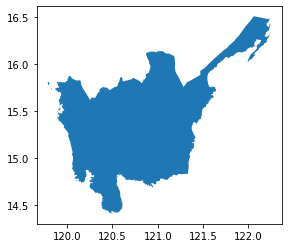region3_gdf.crs  # CRS info

<Geographic 2D CRS: EPSG:4326>
Name: WGS 84
Axis Info [ellipsoidal]:
- Lat[north]: Geodetic latitude (degree)
- Lon[east]: Geodetic longitude (degree)
Area of Use:
- name: World.
- bounds: (-180.0, -90.0, 180.0, 90.0)
Datum: World Geodetic System 1984 ensemble
- Ellipsoid: WGS 84
- Prime Meridian: Greenwich
aoi_total_bounds = region3_gdf.total_bounds
aoi_total_bounds

array([119.7858332,  14.4075257, 122.2429921,  16.5092548])

## Square Grid Generator

### Creating Grids

Create a grid generator with a size of 5,000 m. The units of the grid size are dependent on the projection parameter
of the grid generator. In this case, the default is EPSG:3857.

grids.SquareGridGenerator?

Init signature:
grids.SquareGridGenerator(
cell_size: float,
grid_projection: str = 'EPSG:3857',
boundary: Union[geowrangler.grids.SquareGridBoundary, List[float]] = None,
)
Docstring:      <no docstring>
File:           ~/geowrangler/geowrangler/grids.py
Type:           type
Subclasses:

grid_generator5k = grids.SquareGridGenerator(5_000)  # 5 km x 5 km square cells


Generate square grids

Notice the time taken to grid the multi polygon at 5K resolution

%%time
# slow
grid_gdf5k = grid_generator5k.generate_grid(region3_gdf)

CPU times: user 614 ms, sys: 0 ns, total: 614 ms
Wall time: 617 ms

grid_gdf5k.plot()

<AxesSubplot:>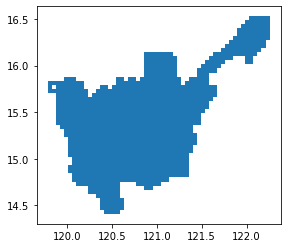Show gridded version of sample geojson file at 5K resolution

length of grid_gdf5k: 1074 row(s)

ax = region3_gdf.plot(facecolor="none", edgecolor="blue")
ax = grid_gdf5k.plot(ax=ax, facecolor="none", edgecolor="green")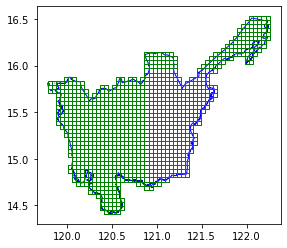In addition to the grid cells, there are 2 extra columns x and y when combined are unique per grid. It can also tell us which grids are adjacent to each other.

grid_gdf5k.head()

x y geometry
0 7 8 POLYGON ((120.10024 14.75528, 120.14516 14.755...
1 6 8 POLYGON ((120.05533 14.75528, 120.10024 14.755...
2 9 8 POLYGON ((120.19008 14.75528, 120.23499 14.755...
3 2 24 POLYGON ((119.87566 15.44910, 119.92058 15.449...
4 2 25 POLYGON ((119.87566 15.49239, 119.92058 15.492...
ax = region3_gdf.plot(facecolor="none", edgecolor="blue")
ax = grid_gdf5k[grid_gdf5k["x"] == 10].plot(ax=ax, facecolor="none", edgecolor="green")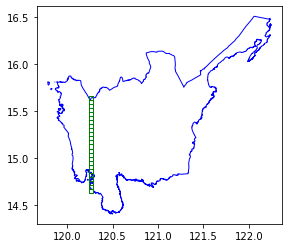### Gridding at a coarser resolution

Let's try the same process but with a bigger grid (15K)

grid_generator15k = grids.SquareGridGenerator(15_000)  # 15 km x 15 km grids


Generate square grids

Notice the time taken to grid the multi polygon at 15K resolution (compared to 5K resolution)

%%time
grid_gdf15k = grid_generator15k.generate_grid(region3_gdf)

CPU times: user 548 ms, sys: 0 ns, total: 548 ms
Wall time: 547 ms

ax = region3_gdf.plot(facecolor="none", edgecolor="blue")
grid_gdf15k.plot(ax=ax, facecolor="none", edgecolor="green")

<AxesSubplot:>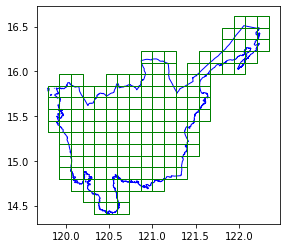Show gridded version of sample geojson file at 15K resolution

length of grid_gdf15k: 154 row(s)


### Reusing boundaries

If you have 2 polygons that are far from each other but wish to have them follow the reference same reference x and y, you can use pass in custom boudaries.

Let's load some grids that are from each other

cell1 = grid_gdf15k.head(1)
cell2 = grid_gdf15k.tail(1)

pd.concat([cell1, cell2])

x y geometry
0 2 2 POLYGON ((120.05533 14.66839, 120.19008 14.668...
153 18 16 POLYGON ((122.21128 16.48548, 122.34603 16.485...
ax = region3_gdf.plot(facecolor="none", edgecolor="red")
ax = grid_gdf15k.plot(ax=ax, facecolor="none", edgecolor="green")
ax = pd.concat([cell1, cell2]).plot(ax=ax)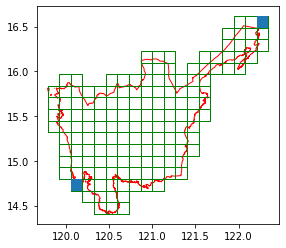grid_generator1k = grids.SquareGridGenerator(1_000, boundary=aoi_total_bounds)

%%time
gridcell1 = grid_generator1k.generate_grid(cell1)

CPU times: user 27.1 ms, sys: 0 ns, total: 27.1 ms
Wall time: 27.8 ms

len(gridcell1)

272
gridcell1.head()

x y geometry
0 29 30 POLYGON ((120.04634 14.66839, 120.05533 14.668...
1 29 31 POLYGON ((120.04634 14.67708, 120.05533 14.677...
2 29 32 POLYGON ((120.04634 14.68577, 120.05533 14.685...
3 29 33 POLYGON ((120.04634 14.69446, 120.05533 14.694...
4 29 34 POLYGON ((120.04634 14.70315, 120.05533 14.703...
ax = gridcell1.plot(facecolor="none", edgecolor="green")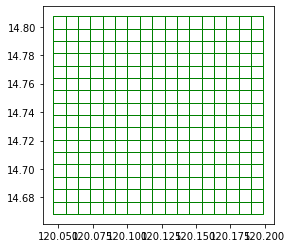%%time
gridcell2 = grid_generator1k.generate_grid(cell2)

CPU times: user 20.2 ms, sys: 0 ns, total: 20.2 ms
Wall time: 18.7 ms

len(gridcell2)

20
gridcell2.head()

x y geometry
0 269 239 POLYGON ((122.20230 16.47686, 122.21128 16.476...
1 269 240 POLYGON ((122.20230 16.48548, 122.21128 16.485...
2 269 241 POLYGON ((122.20230 16.49409, 122.21128 16.494...
3 269 242 POLYGON ((122.20230 16.50270, 122.21128 16.502...
4 270 239 POLYGON ((122.21128 16.47686, 122.22027 16.476...
ax = gridcell2.plot(facecolor="none", edgecolor="red")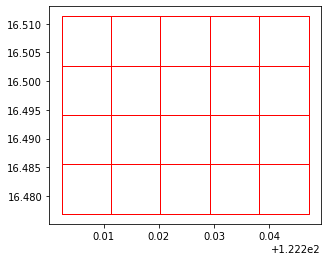ax = region3_gdf.plot(facecolor="none", edgecolor="blue")
ax = gridcell1.plot(ax=ax, color="green")
ax = gridcell2.plot(ax=ax, color="red")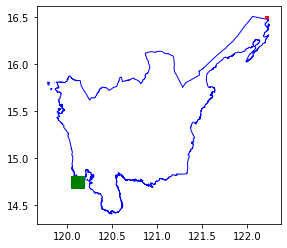## Generating grids for multiple distant areas

If you are using AOIs that are vary far from each other, Grid Generator already optmizes the implementation for you

sparse_aois = grid_gdf15k.iloc[
0:1000:3,
]  # Get areas that far from each other
ax = region3_gdf.plot(facecolor="none", edgecolor="blue")
ax = sparse_aois.plot(ax=ax, facecolor="none", edgecolor="green")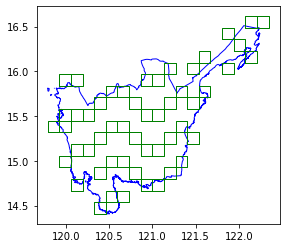sparse_grid = grid_generator1k.generate_grid(sparse_aois)

ax = region3_gdf.plot(facecolor="none", edgecolor="blue")
ax = sparse_grid.plot(ax=ax, facecolor="none", edgecolor="green")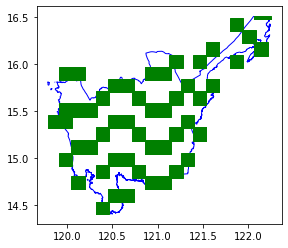It is a bit hard to see the grids so, we get a subset to verify.

ax = sparse_grid.head(1000).plot(facecolor="none", edgecolor="green")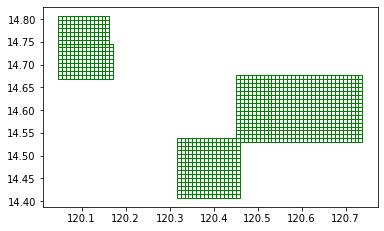## H3 Grid Generator

### Generating grids

h3_generator = grids.H3GridGenerator(resolution=5)

%%time
h3_5_gdf = h3_generator.generate_grid(region3_gdf)

CPU times: user 2.79 s, sys: 6.39 ms, total: 2.8 s
Wall time: 2.81 s

ax = region3_gdf.plot(aspect="equal")
ax = h3_5_gdf.plot(ax=ax, facecolor="none", edgecolor="blue", aspect="equal")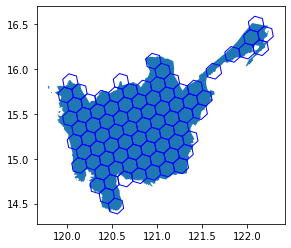### Not exporting geometry

If you do not plan to use use geometry, you can pass return_geometry=False to just get a normal pandas DataFrame with the ids.

h3_generator_no_geom = grids.H3GridGenerator(resolution=5, return_geometry=False)

%%time
h3_region3_no_geom = h3_generator_no_geom.generate_grid(region3_gdf)

CPU times: user 2.75 s, sys: 26.9 ms, total: 2.78 s
Wall time: 2.78 s

len(h3_region3_no_geom)

84
h3_region3_no_geom.head()

hex_id
0 85694103fffffff
1 856941d7fffffff
2 85694e83fffffff
3 85694e93fffffff
4 85694387fffffff

## Bing Tile Grid Generator

### Generating grids

bing_tile_grid_generator = grids.BingTileGridGenerator(12)

%%time
# slow
bing_tile_gdf = bing_tile_grid_generator.generate_grid(region3_gdf)

CPU times: user 7.37 s, sys: 0 ns, total: 7.37 s
Wall time: 7.37 s

ax = region3_gdf.plot(facecolor="none", edgecolor="blue")
ax = bing_tile_gdf.plot(ax=ax, facecolor="none", edgecolor="green")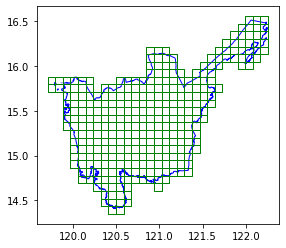### Not exporting geometry

If you do not plan to use use geometry, you can pass return_geometry=False to just get a normal pandas DataFrame with the ids.

bing_tile_grid_generator_no_geom = grids.BingTileGridGenerator(
12, return_geometry=False
)

%%time
# slow
bing_region3_keys = bing_tile_grid_generator_no_geom.generate_grid(region3_gdf)

CPU times: user 7.45 s, sys: 7.19 ms, total: 7.45 s
Wall time: 7.45 s

bing_region3_keys.head()

0 132303030330
1 132303030331
2 132303030313
3 132303012302
4 132303012031

### Converting quadkey to x,y,z format

If you need to convert the quadkey to x,y format, you can pass add_xyz_cols=True to add the x and y columns to the returned dataframe.

bing_tile_grid_generator_add_xyz = grids.BingTileGridGenerator(12, add_xyz_cols=True)

%%time
# slow

CPU times: user 8.85 s, sys: 20 ms, total: 8.87 s

bing_region3_keys.head()